4. 栈（Stack）## 4.0. 什么是栈？• 线性表由若干元素组成，用来存储信息。
• 元素之间有顺序。
• 除了首元素（只有一个直接后继元素）和尾元素（只有一个直接前驱元素）外，其它元素都有且仅有一个直接前驱元素和一个直接后继元素。

• 只能在一端进行操作（增删改查等）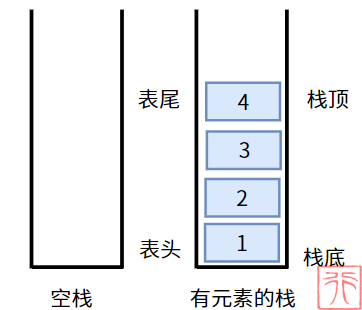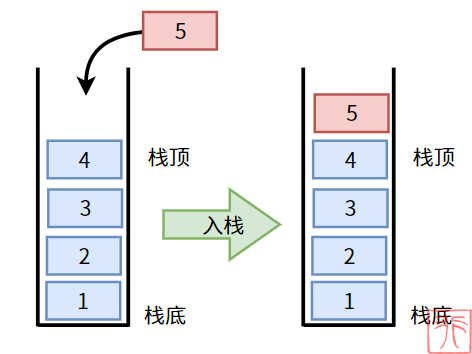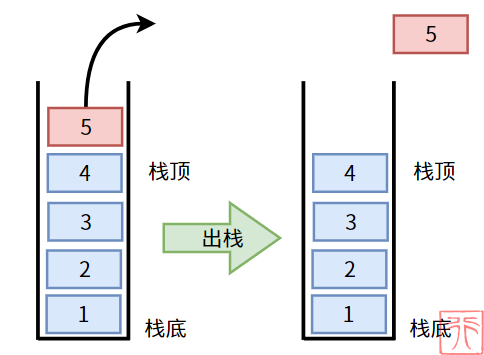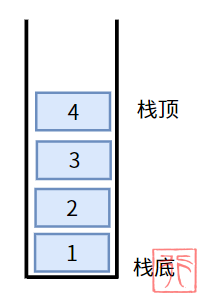## 4.1. 栈的实现思路

### 4.1.0. 数组实现——顺序栈

• 用来存储数据的数组—— `data[]`
• 用来表示线性表的最大存储容量的值——`MAXSIZE`
• 用来标识栈顶元素的栈顶下标—— `top`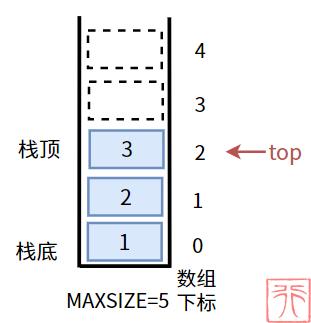``````#define MAXSIZE 5 //栈的最大存储容量

/*顺序栈的结构体*/
typedef struct {
int data[MAXSIZE]; //存储数据的数组
int top; //栈顶下标
}``````

### 4.1.1. 链表实现——链栈

• 链表的基本单元结点 —— `StackNode`
• 结点的数据域—— `data`
• 结点的指针域—— `next`
• 指向链表头的头指针 —— `head`
• 指向栈顶元素的栈顶指针 —— `top`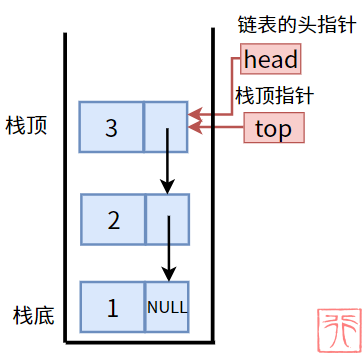``````/*链栈结点的结构体*/
typedef struct StackNode {
int data; //数据域
StackNode *next; //指针域
} StackNode;``````
``````/*栈的结构体*/
typedef struct {
StackNode *top; //栈顶指针
int length; //栈的长度

## 4.2. 栈的状态

### 4.2.0. 顺序栈的状态

【空栈】：栈中没有元素。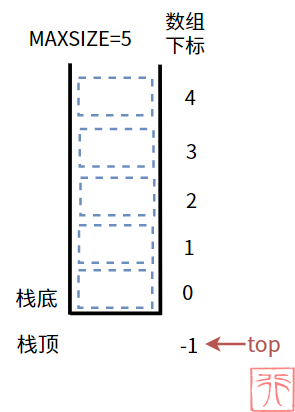【满栈】：栈中元素已满，没有多余容量。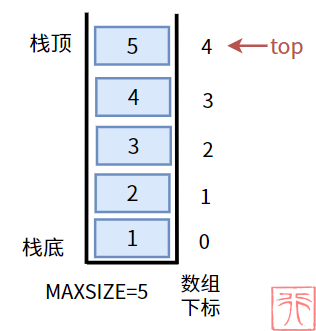【非空非满栈】： 栈不是空栈且容量仍有剩余。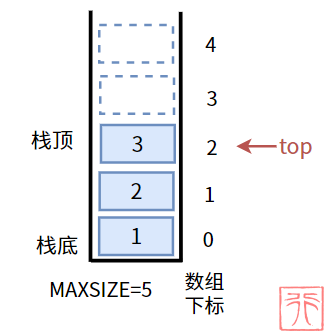### 4.2.1. 链栈的状态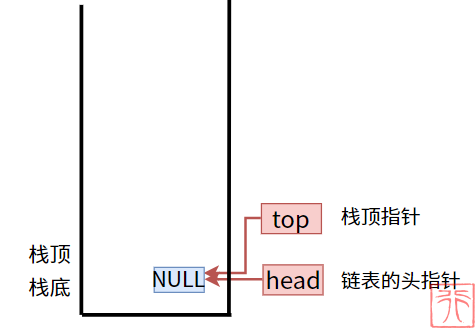## 4.3. 初始化

### 4.3.0. 顺序栈的初始化

``````/**
* 顺序栈的初始化：将栈的栈顶下标置为 -1
* stack: 指向要操作的栈的指针
*/
void init(StackArray *stack)
{
stack->top = -1;
}``````

### 4.3.1. 链栈的初始化

``````/**
* 初始化：将栈顶指针置为 NULL，长度置为 0
* stack: 指向要操作的栈的指针
*/
{
stack->top = NULL;
stack->length = 0;
}``````

## 4.4. 入栈操作

### 4.4.0. 顺序栈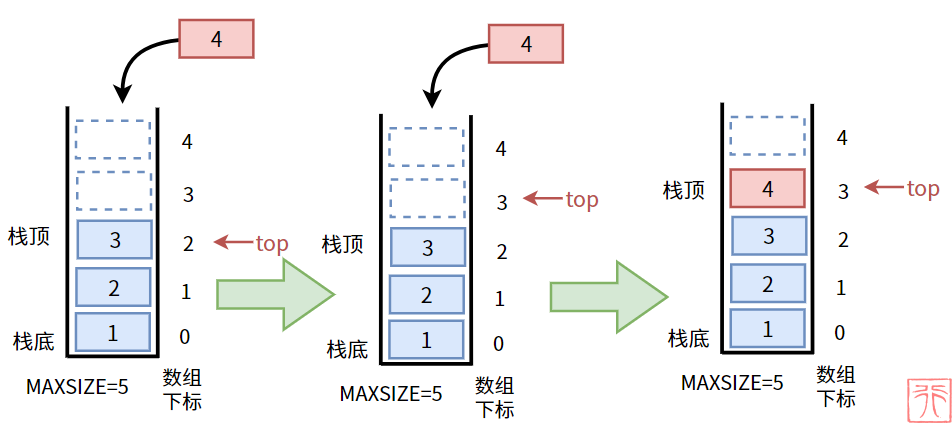``````/**
* 入栈操作
* stack: 指向要操作的栈的指针
* elem: 要入栈的数据
* return: 0失败，1成功
*/
int push(StackArray *stack, int elem)
{
if (stack->top == MAXSIZE - 1) {
printf("栈已满，无法继续入栈。\n");
return 0;
}
stack->top++;
stack->data[stack->top] = elem;
return 1;
}``````

### 4.4.1. 链栈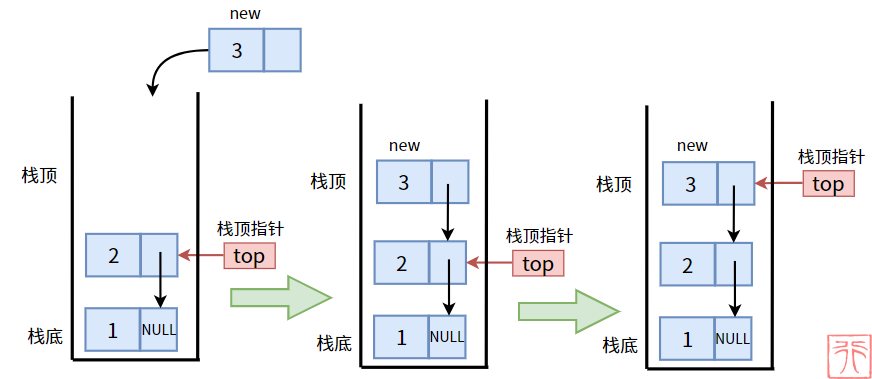``````StackNode *create_node(int elem)
{
StackNode *new = (StackNode *) malloc(sizeof(StackNode));
new->data = elem;
new->next = NULL;
return new;
}

/**
* 入栈操作: 本质是单链表的头插法
* elem: 要入栈的结点的值
*/
{
StackNode *new = create_node(elem);
// 链表的头插法
new->next = stack->top;
stack->top = new;
//栈长度加一
stack->length++;
}``````

## 4.5. 出栈操作

### 4.5.0. 顺序栈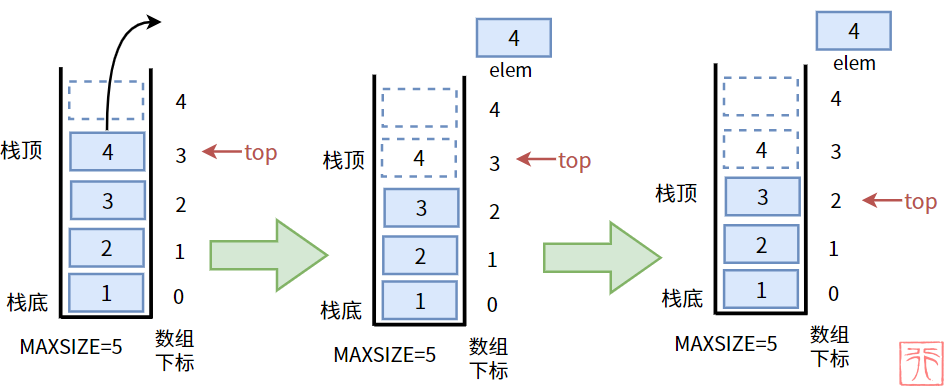``````/**
* 出栈操作
* stack: 指向要操作的栈的指针
* elem: 指向保存变量的指针
* return: 0失败，1成功
*/
int pop(StackArray *stack, int *elem)
{
if (stack->top == -1) {
printf("栈空，无元素可出栈。\n");
return 0;
}
*elem = stack->data[stack->top];
stack->top--;
return 1;
}``````

### 4.5.2. 链栈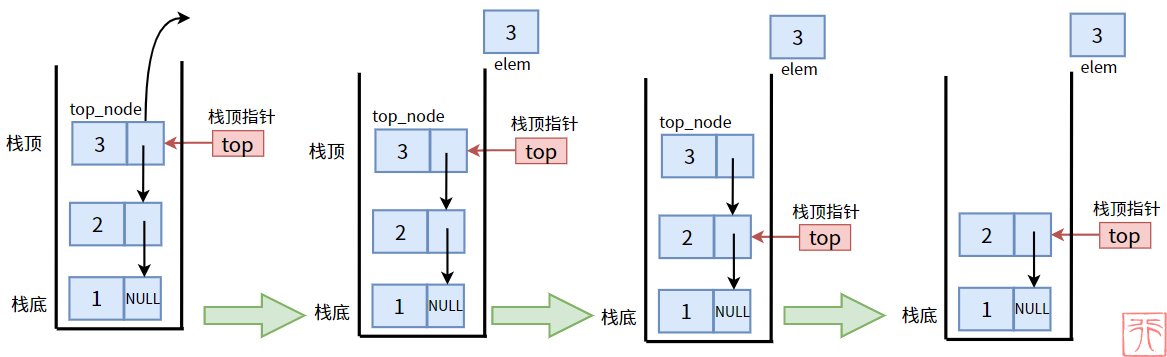``````/**
* 出栈操作: 本质是头删法
* stack: 指向要操作的栈的指针
* elem: 指向保存变量的指针
* return: 0失败，1成功
*/
{
if (stack->length == 0) {
printf("栈空，无元素可出栈。\n");
return 0;
}
// top_node 指向栈顶结点
StackNode *top_node = stack->top;
//保存栈顶结点的值
*elem = top_node->data;
//栈顶指针下移
stack->top = top_node->next;
//释放 top_node
free(top_node);
stack->length--;
return 1;
}``````

## 4.6. 遍历栈

### 4.6.1. 顺序栈

``````/**
* 打印栈
* stack: 要打印的栈
*/
void output(StackArray stack)
{
if (stack.top == -1) {
printf("空栈。\n");
return;
}
for (int i = stack.top; i >= 0; i--) {
printf("%d ", stack.data[i]);
}
printf("\n");
}``````

### 4.6.1. 链栈

``````/**
* 打印栈
* stack: 要打印的栈
*/
{
if (stack->length == 0) {
printf("空栈。\n");
return;
}
StackNode *p = stack->top;
while (p != NULL) {
printf("%d ", p->data);
p = p->next;
}
printf("\n");
}``````22
Lv1
……
16

6

3